# Multivariate time series models¶

Multivariate time series models are designed to capture the dynamic of multiple time series simultaneously and leverage dependencies across these series for more reliable predictions.

The basic requirements in order to use VAR are:

• You need atleast two time series (variables)
• The time series should influence each other.

The vector autoregressive VAR(p) model extends the AR(p) model to k series by creating a system of k equations where each contains p lagged values of all k series. Multivariate time series models allow for lagged values of other time series to affect the target. This effect applies to all series, resulting in complex interactions.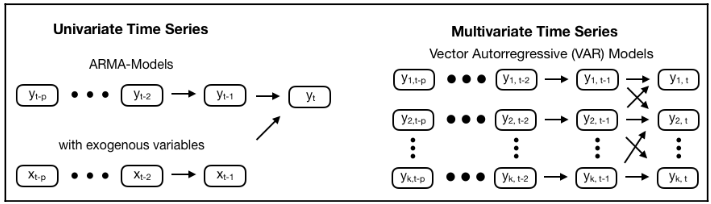In the VAR model, each variable is modeled as a linear combination of past values of itself and the past values of other variables in the system. Since you have multiple time series that influence each other, it is modeled as a system of equations with one equation per variable (time series). A VAR(1) model for k=2 takes the following form:

$$y_{1,t} = c_1 + a_{1,1} y_{1,t-1} + a_{1,2} y_{2,t-1} + \epsilon_{1,t}$$$$y_{1,t} = c_2 + a_{2,1} y_{1,t-1} + a_{2,2} y_{2,t-1} + \epsilon_{1,t}$$

Since the Y terms in the equations are interrelated, the Y’s are considered as endogenous variables, rather than as exogenous predictors.

• VAR(p) models also require stationarity.

# How to use the VAR model for macro fundamentals forecasts¶

## Imports and Settings¶

In :
import os
import sys
import warnings
from datetime import date

import pandas as pd
import numpy as np
from numpy.linalg import LinAlgError
from numpy import cumsum, log, polyfit, sqrt, std, subtract

import matplotlib.pyplot as plt
import matplotlib.transforms as mtransforms
import matplotlib.cm as cm
import seaborn as sns

from scipy.stats import spearmanr, pearsonr
from scipy.stats import probplot, moment

import statsmodels.api as sm
import statsmodels.tsa.api as smt
from statsmodels.tsa.api import VAR, VARMAX
from statsmodels.tsa.stattools import acf, q_stat, adfuller
from statsmodels.graphics.tsaplots import plot_acf, plot_pacf
from statsmodels.tsa.stattools import grangercausalitytests
from statsmodels.stats.stattools import durbin_watson

from sklearn.metrics import mean_squared_error, mean_absolute_error

In :
%matplotlib inline
warnings.filterwarnings('ignore')
sns.set(style='darkgrid', context='notebook', color_codes=True)


## Helper Functions¶

### Hurst Exponent¶

In :
def hurst(ts):
lags = range(2, 100)
tau = [sqrt(std(subtract(ts[lag:], ts[:-lag]))) for lag in lags]
poly = np.polyfit(log(lags), log(tau), 1)
return poly*2.0


### Correlogram Plot¶

In :
def plot_correlogram(x, lags=None, title=None):
lags = min(10, int(len(x)/5)) if lags is None else lags
fig, axes = plt.subplots(nrows=2, ncols=2, figsize=(12, 8))
x.plot(ax=axes)
q_p = np.max(q_stat(acf(x, nlags=lags), len(x)))
axes.text(x=.02, y=.85, s=stats, transform=axes.transAxes)
probplot(x, plot=axes)
mean, var, skew, kurtosis = moment(x, moment=[1, 2, 3, 4])
s = f'Mean: {mean:>12.2f}\nSD: {np.sqrt(var):>16.2f}\nSkew: {skew:12.2f}\nKurtosis:{kurtosis:9.2f}'
axes.text(x=.02, y=.75, s=s, transform=axes.transAxes)
plot_acf(x=x, lags=lags, zero=False, ax=axes)
plot_pacf(x, lags=lags, zero=False, ax=axes)
axes.set_xlabel('Lag')
axes.set_xlabel('Lag')
fig.suptitle(title, fontsize=20)
fig.tight_layout()


### Unit Root Test¶

In :
def test_unit_root(df):


### Durbin Watson Test¶

In :
def durbin_watson_test(df=None, resid=None):
cols, stat = [], []
out = durbin_watson(resid)
for col, val in zip(df.columns, out):
cols.append(col)
stat.append(round(val, 2))
dw_test = pd.DataFrame(stat, index=cols)
return dw_test


We will use monthly data on industrial production and, consumer sentiment provided by the Federal Reserve's data service. We will use the familiar pandas-datareader library to retrieve data from 1972 through 2020:

In :
sent = 'UMCSENT'
df = web.DataReader(['IPGMFN', 'UMCSENT'], 'fred', '1972', '2020-03').dropna()
df.columns = ['ip', 'con_sent']

In :
df.info()

<class 'pandas.core.frame.DataFrame'>
DatetimeIndex: 531 entries, 1972-02-01 to 2020-03-01
Data columns (total 2 columns):
ip          531 non-null float64
con_sent    531 non-null float64
dtypes: float64(2)
memory usage: 12.4 KB


# Plot Series¶

In :
df.plot(subplots=True, figsize=(13,13));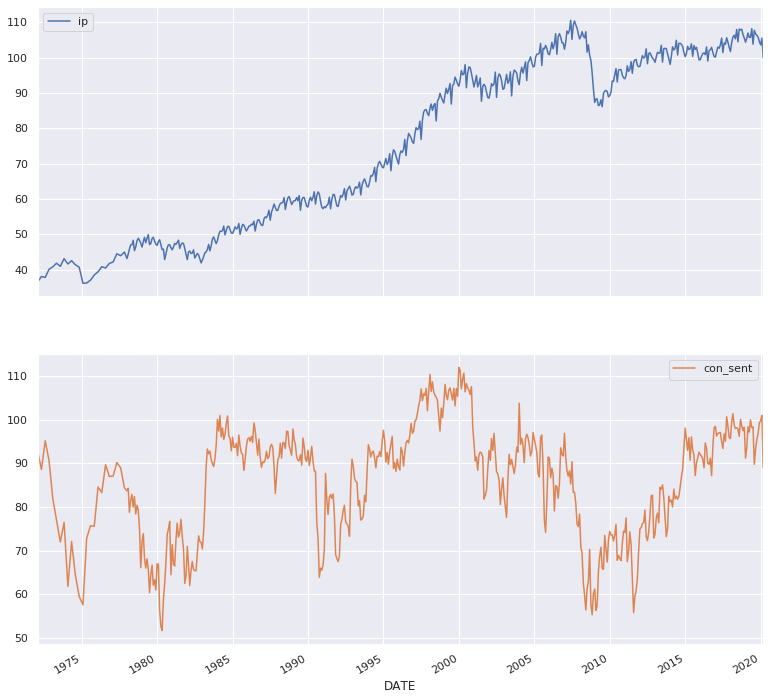# EDA¶

In :
g = sns.clustermap(df.corr(), annot=True)
ax = g.ax_heatmap
bottom, top = ax.get_ylim()
ax.set_ylim(bottom + 0.5, top - 0.5)
plt.title('Pairwise Correlations')
plt.show()

sns.jointplot(x=df.ip.pct_change().dropna(), y=df.con_sent.pct_change().dropna(), kind="reg");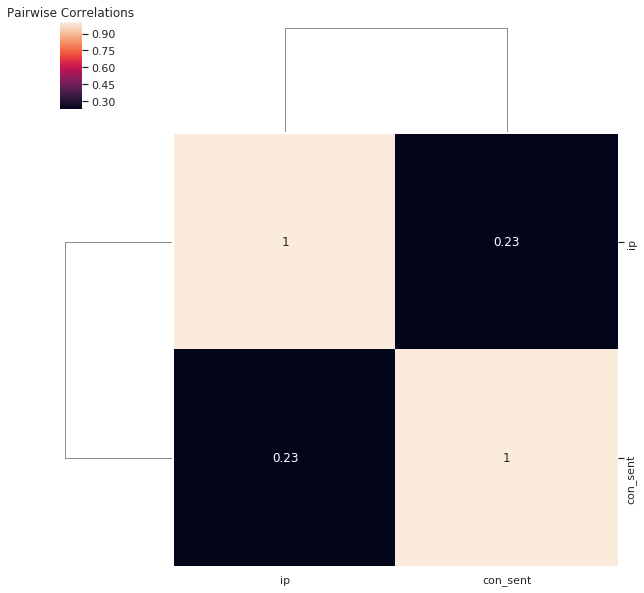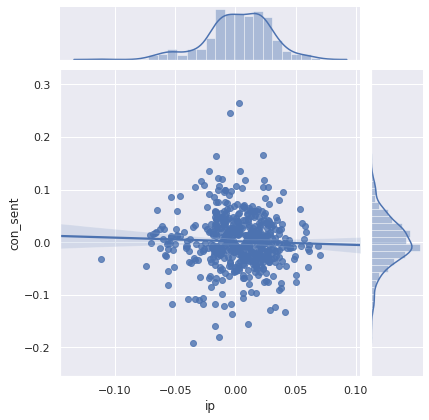The next step in the analysis is to check for causality amongst these series. The Granger’s Causality test and the Cointegration test can help us with that.

# Testing Causation using Granger’s Causality Test¶

The Granger causality test is a statistical hypothesis test for determining whether one time series is useful in forecasting another. Ordinarily, regressions reflect "mere" correlations, but Clive Granger argued that causality in economics could be tested for by measuring the ability to predict the future values of a time series using prior values of another time series. Since the question of "true causality" is deeply philosophical, and because of the post hoc fallacy of assuming that one thing preceding another can be used as a proof of causation, econometricians assert that the Granger test finds only "predictive causality". Rather than testing whether Y causes X, the Granger causality tests whether Y forecasts X.

A time series X is said to Granger-cause Y if it can be shown, usually through a series of t-tests and F-tests on lagged values of X (and with lagged values of Y also included), that those X values provide statistically significant information about future values of Y. The original definition of Granger causality does not account for latent confounding effects and does not capture instantaneous and non-linear causal relationships.

In simpler terms, the past values of time series (X) do not cause the other series (Y). So, if the p-value obtained from the test is lesser than the significance level of 0.05, then, you can safely reject the null hypothesis.

The below code implements the Granger’s Causality test for all possible combinations of each time series in a given dataframe and stores the p-values of each combination in matrix form.

In :
maxlag = 12
test = 'ssr_chi2test'

def grangers_causation_matrix(data, variables, test='ssr_chi2test', verbose=False):
df = pd.DataFrame(np.zeros((len(variables), len(variables))), columns=variables, index=variables)
for c in df.columns:
for r in df.index:
test_result = grangercausalitytests(data[[r, c]], maxlag=maxlag, verbose=False)
p_values = [round(test_result[i+1][test],4) for i in range(maxlag)]
if verbose: print(f'Y = {r}, X = {c}, P Values = {p_values}')
min_p_value = np.min(p_values)
df.loc[r, c] = min_p_value
df.columns = [var + '_x' for var in variables]
df.index = [var + '_y' for var in variables]
return df

In :
grangers_df = grangers_causation_matrix(df, variables = df.columns)

plt.figure(figsize=(8,5))
sns.heatmap(grangers_df, annot=True)
b, t = plt.ylim()
b += 0.5
t -= 0.5
plt.ylim(b, t)
plt.show()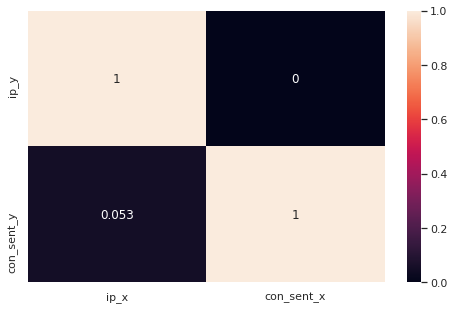## Find Significant Pairs in a p-value matrix¶

In :
pairs = grangers_df.unstack()
pairs = pairs.sort_values(kind="quicksort")

print("Significan Pairs")

Significan Pairs
con_sent_x  ip_y    0.0
dtype: float64


Looking at the P-Values in the above table, you can pretty much observe that most the variables (time series) in the system are interchangeably causing each other. This makes this system of multi time series a good candidate for using VAR models to forecast.

# Cointegration Test¶

Cointegration test helps to establish the presence of a statistically significant connection between two or more time series. Cointegration is a statistical property of two or more time-series variables which indicates if a linear combination of the variables is stationary.

When two or more time series are cointegrated, it means they have a long run, statistically significant relationship.

This is the basic premise on which Vector Autoregression(VAR) models is based on. So it is common to implement the cointegration test before starting to build VAR models.

Implementation of the cointegration test in python’s statsmodels can see below:

In :
def find_cointegrated_pairs(dataframe, critial_level = 0.05):
n = dataframe.shape
pvalue_matrix = np.ones((n, n))
keys = dataframe.columns
pairs = []
for i in range(n):
for j in range(i+1, n):
series1 = dataframe[keys[i]]
series2 = dataframe[keys[j]]
result = sm.tsa.stattools.coint(series1, series2)
pvalue = result
pvalue_matrix[i, j] = pvalue
if pvalue < critial_level:
pairs.append((keys[i], keys[j], pvalue))
return pvalue_matrix, pairs

In :
pvalue_matrix, pairs = find_cointegrated_pairs(df)
coint_pvalue_matrix_df = pd.DataFrame(pvalue_matrix)

g = sns.clustermap(coint_pvalue_matrix_df, xticklabels=df.columns,yticklabels=df.columns, annot=True,
figsize=(8, 8))
plt.title('Cointegration P-value Matrix')
ax = g.ax_heatmap
bottom, top = ax.get_ylim()
ax.set_ylim(bottom + 0.5, top - 0.5)
plt.show()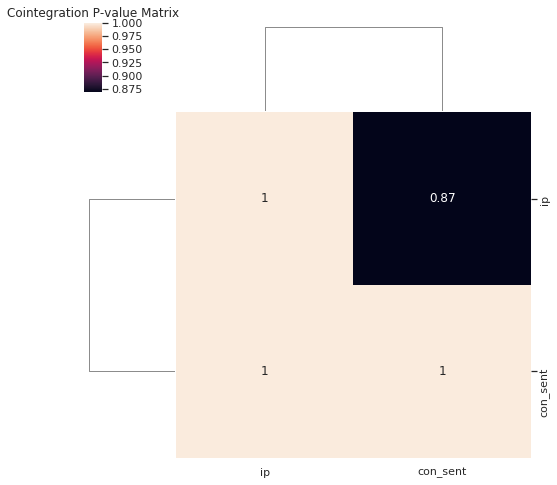In :
pairs

Out:
[]

Pair is not significant

# Check for Stationarity¶

Since the VAR model requires the time series you want to forecast to be stationary, it is customary to check all the time series in the system for stationarity.

A stationary time series is one whose mean and variance does not change over time.

So, how to test for stationarity?

There is a suite of tests called unit-root tests. The popular ones are:

• Augmented Dickey-Fuller Test (ADF Test)
• KPSS test
• Philip-Perron test

Let’s implement the ADF Test by using our plot_correlogram() function which retuns a p-value for the ADF test.

In :
for i in df.columns:
plot_correlogram(df[i], lags=100, title=f'{i}')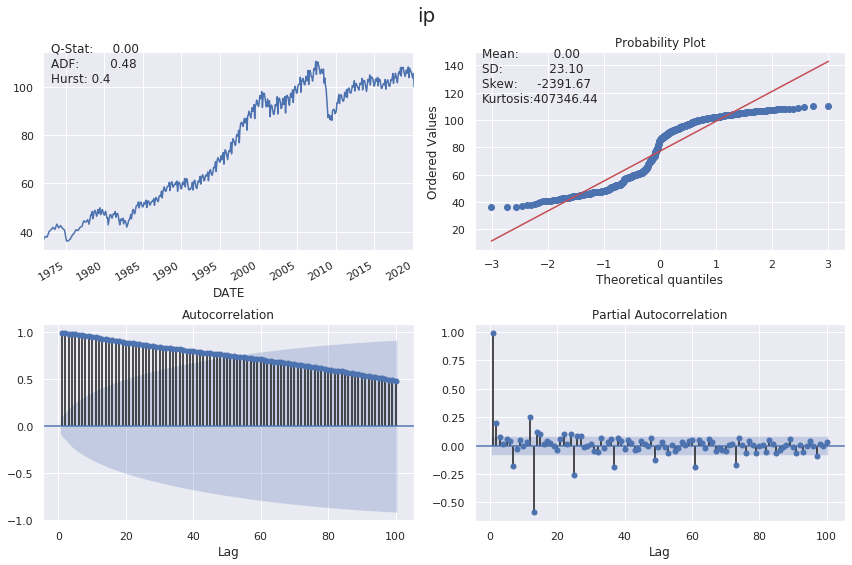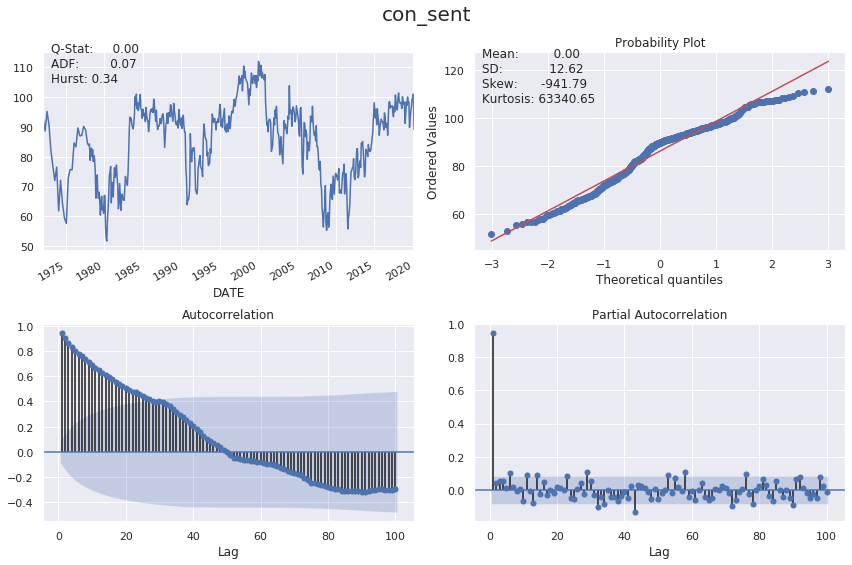## Stationarity Transform¶

Log-transforming the industrial production and differencing both series using lag 12 yields stationary results:

In :
df_transformed = pd.DataFrame({'ip': np.log(df.ip).diff(12),
'con_sent': df.con_sent.diff(12)}).dropna()


## Inspect Correlograms¶

In :
for i in df_transformed.columns:
plot_correlogram(df_transformed[i], lags=100, title=f'{i}_log_diff')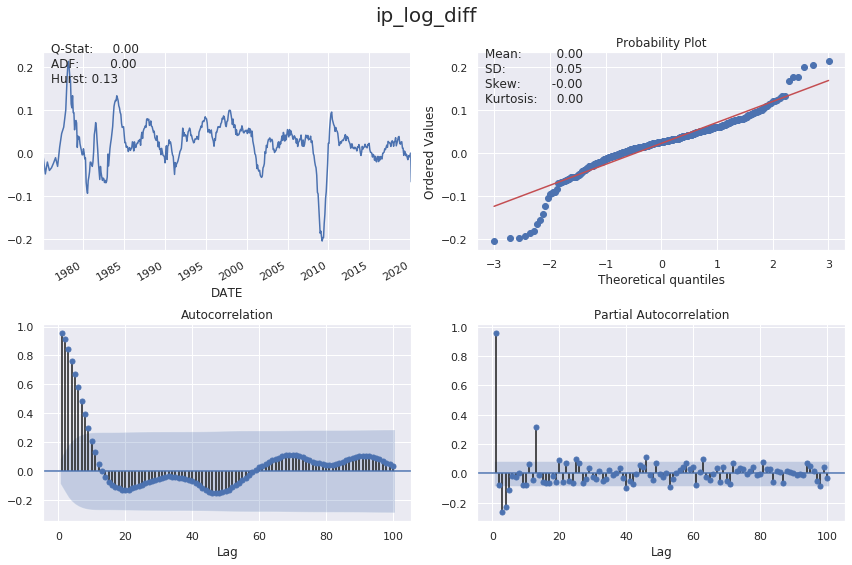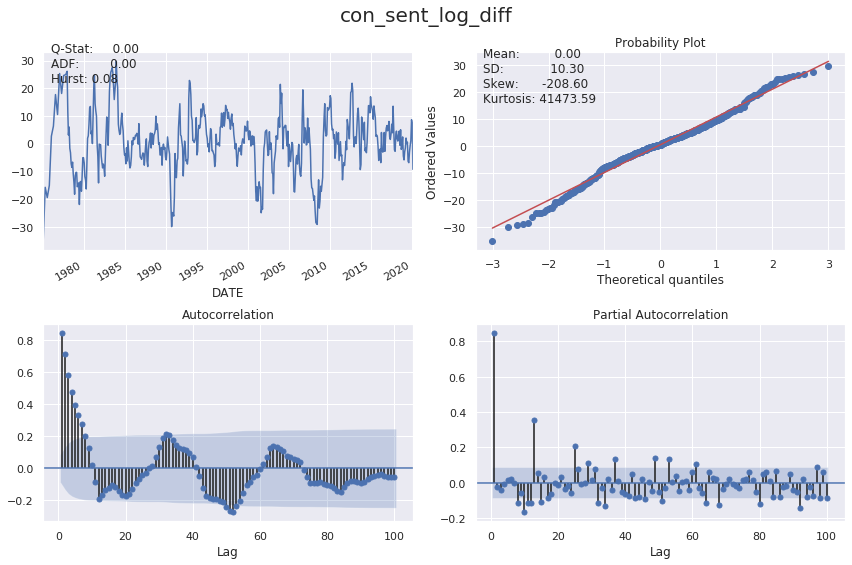In :
test_unit_root(df_transformed)

Out:
p-value
ip 0.04%
con_sent 0.00%

All the series are now stationary.

## Split the Series into Training and Testing Data¶

In :
split = int(len(df) * 0.91)
df_train, df_test = df_transformed.iloc[:split], df_transformed.iloc[split:]
print(df_train.info())
print(df_test.info())

<class 'pandas.core.frame.DataFrame'>
DatetimeIndex: 483 entries, 1975-02-01 to 2017-03-01
Data columns (total 2 columns):
ip          483 non-null float64
con_sent    483 non-null float64
dtypes: float64(2)
memory usage: 11.3 KB
None
<class 'pandas.core.frame.DataFrame'>
DatetimeIndex: 36 entries, 2017-04-01 to 2020-03-01
Data columns (total 2 columns):
ip          36 non-null float64
con_sent    36 non-null float64
dtypes: float64(2)
memory usage: 864.0 bytes
None


### How to Select the Order (P) of VAR model¶

To select the right order of the VAR model, we iteratively fit increasing orders of VAR model and pick the order that gives a model with least BIC.

In :
test_results = {}

for p in range(5):
for q in range(5):
if p == 0 and q == 0:
continue

print(f'Testing Order: p = {p}, q = {q}')
convergence_error = stationarity_error = 0

try:
model = VARMAX(df_train, order=(p,q), trend='n')
model_result = model.fit(maxiter=1000, disp=False)

except LinAlgError:
convergence_error += 1

except ValueError:
stationarity_error += 1

print('\nAIC:', model_result.aic)
print('BIC:', model_result.bic)
print('HQIC:', model_result.hqic)
print('------------------------')

test_results[(p, q)] = [model_result.aic,
model_result.bic,
convergence_error,
stationarity_error]

Testing Order: p = 0, q = 1

AIC: 1371.7385572753833
BIC: 1400.9986738509513
HQIC: 1383.237050802628
------------------------
Testing Order: p = 0, q = 2

AIC: 1135.7477512608878
BIC: 1181.727934451066
HQIC: 1153.8168125179864
------------------------
Testing Order: p = 0, q = 3

AIC: 1275.252227239983
BIC: 1337.9524770447717
HQIC: 1299.8918562269357
------------------------
Testing Order: p = 0, q = 4

AIC: 1029.4102305196916
BIC: 1108.8305469390905
HQIC: 1060.6204272364985
------------------------
Testing Order: p = 1, q = 0

AIC: 161.69557649494536
BIC: 190.95569307051335
HQIC: 173.19407002218995
------------------------
Testing Order: p = 1, q = 1

AIC: 163.52700208187827
BIC: 209.50718527205657
HQIC: 181.59606333897693
------------------------
Testing Order: p = 1, q = 2

AIC: 144.24090067444996
BIC: 206.94115047923856
HQIC: 168.88052966140268
------------------------
Testing Order: p = 1, q = 3

AIC: 141.9579187040034
BIC: 221.37823512340225
HQIC: 173.16811542081015
------------------------
Testing Order: p = 1, q = 4

AIC: 141.10263753476892
BIC: 237.2430205687781
HQIC: 178.88340198142976
------------------------
Testing Order: p = 2, q = 0

AIC: 162.81149410021015
BIC: 208.79167729038846
HQIC: 180.88055535730882
------------------------
Testing Order: p = 2, q = 1

AIC: 145.01807832127838
BIC: 207.71832812606698
HQIC: 169.6577073082311
------------------------
Testing Order: p = 2, q = 2

AIC: 138.27208896193343
BIC: 217.6924053813323
HQIC: 169.4822856787402
------------------------
Testing Order: p = 2, q = 3

AIC: 148.56213646391654
BIC: 244.70251949792572
HQIC: 186.34290091057736
------------------------
Testing Order: p = 2, q = 4

AIC: 149.56204041936866
BIC: 262.4224900679881
HQIC: 193.91337259588352
------------------------
Testing Order: p = 3, q = 0

AIC: 139.30589052036888
BIC: 202.00614032515745
HQIC: 163.9455195073216
------------------------
Testing Order: p = 3, q = 1

AIC: 143.25360935615774
BIC: 222.6739257755566
HQIC: 174.4638060729645
------------------------
Testing Order: p = 3, q = 2

AIC: 153.0810039182775
BIC: 249.22138695228668
HQIC: 190.8617683649383
------------------------
Testing Order: p = 3, q = 3

AIC: 152.44187546543859
BIC: 265.302325114058
HQIC: 196.79320764195347
------------------------
Testing Order: p = 3, q = 4

AIC: 153.81168334895472
BIC: 283.39219961218447
HQIC: 204.73358325532365
------------------------
Testing Order: p = 4, q = 0

AIC: 135.22251541888454
BIC: 214.64283183828343
HQIC: 166.4327121356913
------------------------
Testing Order: p = 4, q = 1

AIC: 142.16620562373652
BIC: 238.3065886577457
HQIC: 179.94697007039736
------------------------
Testing Order: p = 4, q = 2

AIC: 151.3080900618475
BIC: 264.168539710467
HQIC: 195.6594222383624
------------------------
Testing Order: p = 4, q = 3

AIC: 157.9605559838041
BIC: 287.54107224703387
HQIC: 208.88245589017305
------------------------
Testing Order: p = 4, q = 4

AIC: 156.7242040017988
BIC: 303.02478687963884
HQIC: 214.21667163802175
------------------------

In :
test_results = pd.DataFrame(test_results).T
test_results.columns = ['AIC', 'BIC', 'convergence', 'stationarity']
test_results.index.names = ['p', 'q']
test_results.info()

<class 'pandas.core.frame.DataFrame'>
MultiIndex: 24 entries, (0, 1) to (4, 4)
Data columns (total 4 columns):
AIC             24 non-null float64
BIC             24 non-null float64
convergence     24 non-null float64
stationarity    24 non-null float64
dtypes: float64(4)
memory usage: 1012.0 bytes


#### We aim to minimize BIC:¶

In :
sns.heatmap(test_results.BIC.unstack(), fmt='.2f', annot=True, cmap='Blues_r')
b, t = plt.ylim()
b += 0.5
t -= 0.5
plt.ylim(b, t)
plt.show()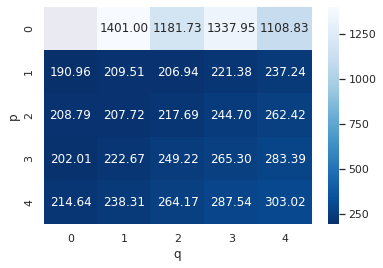In :
test_results.sort_values('BIC').head()

Out:
AIC BIC convergence stationarity
p q
1 0 161.695576 190.955693 0.0 0.0
3 0 139.305891 202.006140 0.0 0.0
1 2 144.240901 206.941150 0.0 0.0
2 1 145.018078 207.718328 0.0 0.0
0 162.811494 208.791677 0.0 0.0

# Estimating with the best VAR Model¶

We will estimate a VAR(1,0) model using the statsmodels VARMAX implementation (which allows for optional exogenous variables) with a no trend using the first 164 observations. The output contains the coefficients for both time series equations.

In :
model = VARMAX(df_train, order=(1,0), trend='n').fit(maxiter=1000)
model.summary()

Out:
Dep. Variable: No. Observations: ['ip', 'con_sent'] 483 VAR(1) -73.848 Sun, 26 Apr 2020 161.696 19:14:21 190.956 0 173.194 - 483 opg
Ljung-Box (Q): Jarque-Bera (JB): 131.02, 172.32 145.74, 16.20 0.00, 0.00 0.00, 0.00 0.45, 1.11 0.18, 0.20 0.00, 0.52 5.67, 3.80
coef std err z P>|z| [0.025 0.975] 0.9397 0.009 107.585 0.000 0.923 0.957 0.0006 5.24e-05 10.948 0.000 0.000 0.001
coef std err z P>|z| [0.025 0.975] -8.4967 4.208 -2.019 0.043 -16.743 -0.250 0.8681 0.018 48.206 0.000 0.833 0.903
coef std err z P>|z| [0.025 0.975] 0.0128 0.000 42.008 0.000 0.012 0.013 0.0764 0.222 0.344 0.731 -0.359 0.512 5.2497 0.141 37.186 0.000 4.973 5.526

Warnings:
 Covariance matrix calculated using the outer product of gradients (complex-step).

## Plot Diagnostics¶

statsmodels provides diagnostic plots to check whether the residuals meet the white noise assumptions, which are not exactly met in this simple case:

Industrial Production

In :
model.plot_diagnostics(variable=0, figsize=(13,8), lags=24)
plt.gcf().suptitle('Industrial Production - Diagnostics', fontsize=20)
plt.tight_layout()